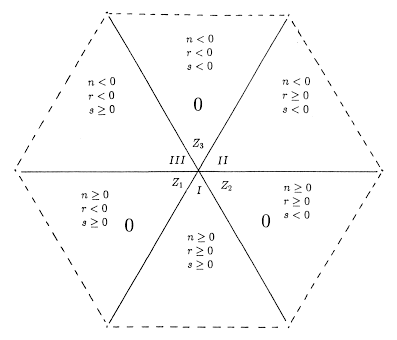## 1. The Construction of the Pascal Hexagon

Usually the binomial coefficient

 æ ç è n r ö ÷ ø
is defined for n ³ 0, n ³r ³ 0, and is then given by

 æ ç è n r ö ÷ ø = n! r!(n-r)! .                                     (1.1)

 æ ç è n r s ö ÷ ø
for
 æ ç è n r ö ÷ ø
with the understanding that
 r + s = n.                                        (1.2)

 æ ç è n r ö ÷ ø = n! r!s! .                                    (1.3)
if n ³ 0, r ³ 0, s ³ 0.

Thus (1.3) may be regarded as giving the value of

 æ ç è n r s ö ÷ ø
in one sextant of the Pascal Hexagon, naturally called the positive triangle (and well-known as the Pascal Triangle). We note that, with n ³ 0,
 æ ç è n r s ö ÷ ø
is the coefficient of arbs in the binomial expansion of (a+b)n. This remark gives us the clue as to how to define over the entire hexagon, that is, for arbitrary integer values of n, r, s. Obviously we should define

 æ ç è n r s ö ÷ ø = 0 (1.4)

if n ³ 0 and r or s negative.

 if |a| < |b|, then (a+b)n = å r ³ 0 æ ç è n r s ö ÷ ø arbs where æ ç è n r s ö ÷ ø = (-1)r æ ç è -s-1 r,-n-1 ö ÷ ø (1.5)

 if |a| > |b|, then (a+b)n = å s ³ 0 æ ç è n r s ö ÷ ø arbs where æ ç è n r s ö ÷ ø = (-1)s æ ç è -r-1 -n-1, s ö ÷ ø (1.6)

Thus we define

 æ ç è n r s ö ÷ ø = (-1)r æ ç è -s-1 r,-n-1 ö ÷ ø if    n<0, r ³ 0, s < 0.            (1.7)

 æ ç è n r s ö ÷ ø = (-1)s æ ç è -r-1 -n-1, s ö ÷ ø if    n<0, r < 0, s ³ 0.            (1.8)

We complete the Pascal Hexagon in the obvious way by setting

 æ ç è n r s ö ÷ ø = 0 if    n<0, r < 0, s < 0.            (1.9)

We note the following properties of

 æ ç è n r s ö ÷ ø
so defined.

Symmetry Identity

 æ ç è n r s ö ÷ ø = æ ç è n s r ö ÷ ø (1.10)

Pascal Identity

 æ ç è n r     s ö ÷ ø = æ ç è n-1 r-1     s ö ÷ ø + æ ç è n-1 r     s-1 ö ÷ ø (1.11)

except that
 æ ç è 0 0     0 ö ÷ ø ¹ æ ç è -1 -1     0 ö ÷ ø + æ ç è -1 0     -1 ö ÷ ø (1.12)

Of course, the exception here is not bothersome as it is concerned with the only occurrence of the Pascal relationship which is not contained in a single sextant of the hexagon.

Certain qualitative features of the Pascal Hexagon are shown in Figure 1(a). Notice the directions in which n, r and s are constant (Figure 1(b)) and the natural division of the hexagon into 6 triangular sextants. Three of these are non-zero sextants, labelled I, II, III, in which every entry is non-zero; and three are zero sextants, labelled Z1, Z2, Z3,  in which every entry is zero. Note that the frontier lines between sextants are all regarded as belonging to the non-zero sextants they abut, except that the center point belongs to I.Figure 1a: Remember, r + s = n.Figure 1b: Directions in which n, r, s are constant.

We suggest you take a few minutes to look at the portion of the Pascal Hexagon we give in Figure 1. In particular, compare the numbers (with their signs) that occur in the northeast and northwest sectors with those that occur in the ordinary Pascal Triangle. You may then see a very easy way to build the hexagon out of the Pascal Triangle.Figure 2: The Pascal Hexagon for 10³n, r, s ³ -11. Note that we have slightly displaced the frontier lines between sextants in order to be able to display the non-zero values along these lines.

There are fascinating patterns within each non-zero sextant of the Pascal Hexagon. However, we are principally concerned in this paper with patterns which cross the sextants of the Pascal Hexagon.

1 If the entries for r and s are such that confusion might result we will insert a comma and write
 æ ç è n r,s ö ÷ ø